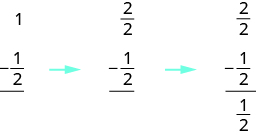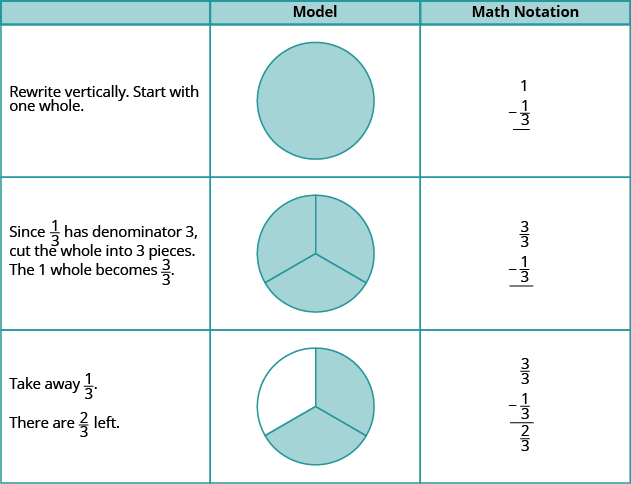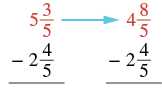Subtracting Mixed Numbers with a Common Denominator

Learning Outcomes

• Use a model to subtract mixed numbers with like denominators
• Subtract mixed numbers with like denominators using two different methods

Let’s think of pizzas again to model subtraction of mixed numbers with a common denominator. Suppose you just baked a whole pizza and want to give your brother half of the pizza. What do you have to do to the pizza to give him half? You have to cut it into at least two pieces. Then you can give him half.

We will use fraction circles (pizzas!) to help us visualize the process.Algebraically, you would write:Example

Use a model to subtract: $1-\Large\frac{1}{3}$.

Solution:Try It

What if we start with more than one whole? Let’s find out.

Example

Use a model to subtract: $2-\Large\frac{3}{4}$.

Try It

In the next example, we’ll subtract more than one whole.

Example

Use a model to subtract: $2 - 1\Large\frac{2}{5}$.

try It

What if you start with a mixed number and need to subtract a fraction? Think about this situation: You need to put three quarters in a parking meter, but you have only a $1$ bill and one quarter. What could you do? You could change the dollar bill into $4$ quarters. The value of $4$ quarters is the same as one dollar bill, but the $4$ quarters are more useful for the parking meter. Now, instead of having a $1$ bill and one quarter, you have $5$ quarters and can put $3$ quarters in the meter.

This models what happens when we subtract a fraction from a mixed number. We subtracted three quarters from one dollar and one quarter.

We can also model this using fraction circles, much like we did for addition of mixed numbers.

Example

Use a model to subtract: $1\Large\frac{1}{4}-\Large\frac{3}{4}$

Try It

Use a model to subtract. Draw a picture to illustrate or model your answer.

$1\Large\frac{1}{3}-\Large\frac{2}{3}$

Use a model to subtract. Draw a picture to illustrate your model.
$1\Large\frac{1}{5}-\Large\frac{4}{5}$

Subtract mixed numbers with like denominators

Now we will subtract mixed numbers without using a model. But it may help to picture the model in your mind as you read the steps.

Subtract mixed numbers with common denominators

1. Rewrite the problem in vertical form.
2. Compare the two fractions.
• If the top fraction is larger than the bottom fraction, go to Step 3.
• If not, in the top mixed number, take one whole and add it to the fraction part, making a mixed number with an improper fraction.
3. Subtract the fractions.
4. Subtract the whole numbers.
5. Simplify, if possible.

Example

Find the difference: $5\Large\frac{3}{5}-\normalsize2\Large\frac{4}{5}$

Solution:

 $5\Large\frac{3}{5}-\normalsize2\Large\frac{4}{5}$ Rewrite the problem in vertical form.Since $\Large\frac{3}{5}$ is less than $\Large\frac{4}{5}$ , take 1 from the 5 and add it to the $\Large\frac{3}{5}:\left(\Large\frac{5}{5}+\Large\frac{3}{5}=\Large\frac{8}{5}\right)$Subtract the fractions.Subtract the whole parts. The result is in simplest form.Since the problem was given with mixed numbers, we leave the result as mixed numbers.

Try It

Watch the following video to see two more examples of how to subtract mixed numbers with like denominators.

Just as we did with addition, we could subtract mixed numbers by converting them first to improper fractions. We should write the answer in the form it was given, so if we are given mixed numbers to subtract we will write the answer as a mixed number.

Subtract mixed numbers with common denominators as improper fractions

Step 1. Rewrite the mixed numbers as improper fractions.
Step 2. Subtract the numerators.
Step 3. Write the answer as a mixed number, simplifying the fraction part, if possible.

Example

Find the difference by converting to improper fractions:
$9\Large\frac{6}{11}-\normalsize7\Large\frac{10}{11}$